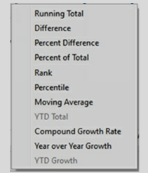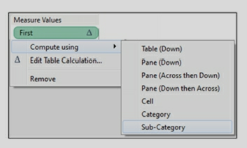##### Follow Us :
>>>>>>Tableau Interview Questions
##### What are Quick Table calculations in Tableau?
Tableau provides some pre-defined Table calculations called Quick Table calculations.

Quick Table calculations also work on returned result set and are created by right-clicking on the measure pill on the view canvas and selecting “Quick Table Calculation” The types of quick calculations available are##### What is Addressing and Partitioning of Data?
Tableau internally assigns all fields in the dataset to be addressing or partitioning. Partitioning field partitions data into separate individual sections. Calculations are applied to these sections.

Addressing field provides the direction in which the calculation will take place.

Exercise: Use Tableau sample data source Sample- Superstore.xls located under \My Tableau Repository\Data sources.
Use the Orders datasheet.
Place Order Date on the Rows, drill down to Quarters. Double click on Sales. Create a Table calculation for “Running Total”.  After the visualization is created, navigate to Sum(Sales) and select edit Table calculation.
Under Running Along, choose the option for Advanced and see which columns are used for Partitioning and Addressing. Switch the columns around to see the changes in the output. .
##### What is First (), Last () and Index () functions in Tableau?
Refer to the Partitioning field’s definition in the previous question.
First, Last and Index Table calculation functions provide information about the position of a data element within a partition.

First() – returns the number of rows from the current row to the first row in the partition. It is a negative number.

Last () – returns the number of rows from the current row to the last row in the partition. It is a positive number.

Index() – returns the index of the current row in the partition. The first-row index starts with

1. Exercise: Create an example to understand First, Last and Index functions.
Place Category on the Rows and drill down to Sub-Category. Place Sales on the Columns. Create calculations for First(), Last(), and Index() and place them on the table in the view canvas.
Observe the output.
Navigate to the function pills on the left under Marks and use Compute using to change the partitioning fields and see the difference in your output.##### What are LOD (Level of Detail) calculations in Tableau?
LOD calculations compute aggregation that is outside the “level of detail” of the view.

LOD expression has a specific syntax and works with keywords such as Include, Exclude, and Fixed. LOD calculation follows the below syntax

{Keyword [Dim1],[Dim2]: Sum(Measure field)}.
##### Explain keywords in LOD expressions?
LOD expressions have the following keywords

a) Fixed computes the value using the mentioned dimensions ignoring the dimension in the view

b) Include computes the value using the mentioned dimension in addition to the dimensions in the view

c) Exclude will ignore the mentioned dimension. It ignores the dimension even if it is used in the view.

d) If no dimension or Keyword is specified then the expression is “Table scoped” which is the complete aggregate of the data. {Sum(Sales)}

e) Only field names can be used in the Dimension declaration. Calculated fields can be used but not the calculation expression. Sets, parameters, or Table calculations are not allowed.

f) The LOD expressions that use Exclude or Include always result in measures. These results cannot be binned.

g) Fixed can be an expression or a dimension based on the aggregation used. If the aggregate expression results in a measure then the overall expression will be a measure. If aggregation such as string, Boolean, or Date types results in dimension then the overall expression will be a dimension. The fixed expression can be converted to a dimension or a measure.

h) Numeric Fixed LOD expressions can be binned unless the aggregate expression is a date.